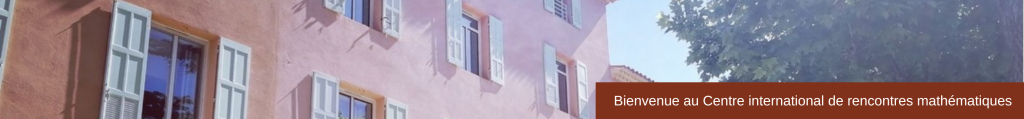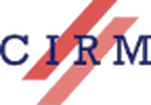RESEARCH IN PAIRS

Associated Varieties of Affine Vertex Algebras and Class S Theory
August 1-12, 2016
 In the thirty years that have passed since they were introduced  by Borcherds, vertex algebras have turned out to be extremely useful in many areas of mathematics, such as algebraic geometry,  the  theory  of finite groups, modular functions, topology, integrable  system,  and combinatorics. The theory of vertex algebras also serves as the rigorous mathematical foun- dation for two-dimensional conformal field theory and string  theory, extensively studied  by physicists.  The notion of vertex algebras was extended  by Beilinson and Drinfeld to chiral algebras, which served as a foundation  of the celebrated  geometric Langlands program. We plan to explore yet another  perspective of vertex algebras. More precisely, this  project  follows our previous  works [Arakawa-Moreau, Joseph ideals and lisse minimal W-algebras, to appear in J. of Inst. Math. Jussieu] and [Arakawa-Moreau, Sheets and associated varieties of affine vertex algebras, preprint].The first article has surprinsgly raised the interest  of some physicists (like Nishinaka, Tachikawa, Rastelli, etc.), thus opening new prospects for applications of vertex algebras, in particular in connection with class S  theory, which is a rich class of four-dimensional field theories with N  = 2 supersymmetry. The class S theory provides numerous interesting questions. In particular it gives conjectural relations between vertex algebras and symplectic varieties.  We wish to understand these interesting relations  during our stay. We also plan to study some conjectures on associated varieties  to affine vertex algebras, started  in our previous works. Participants Tomoyuki Arakawa (Kyoto University)Anne Moreau (Université de Poitiers) Sponsor